# Atoms and Elements

## Atoms

You will need to know:

The subatomic particles of an atom.

The relative charge and mass of each subatomic particle.

All matter is made of atoms. Scientists use to think that atoms are the smallest things in existence, but now they know otherwise.

Atoms are very small particles made up of a electrons orbiting a nucleus. The nucleus is made out of protons and neutrons.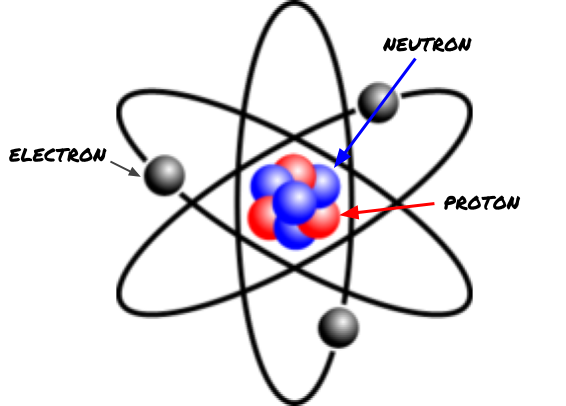Protons, Neutrons and Electrons are called subatomic particles. ‘Subatomic’ means ‘inside the atom’.

It is VERY important to remember the relative masses and __charges __for each subatomic particle. So you should find this table very useful__NOTE: __These masses and charges are relative to an atom of Carbon. This is because the actual mass and charge of an atom are VERY small, so we say how big they are compared to something else that is very small. More on this in the Isotopes section.

All atoms have the same amount of protons and electrons (so if there are 5 protons in the nucleus, there will be 5 electrons orbiting). This means that the charge cancels out so that an atom has 0 overall charge.

Example Question:

What is the overall relative charge and mass of the atom shown in the picture above?

Relative charge: 0

Relative mass: 7

Explanation:

Since there are 3 protons (+3 charge altogether), 3 electrons (-3 charge altogether) and 4 neutrons (0 charge) then these all added together is: +3 -3 = 0

Since there are 4 neutrons (+4 mass all together), 3 protons (+3 mass altogether) and 3 electrons (0 mass) then the overall relative mass is: 4+3 = 7

## Elements

You will need to know:

What an element is.

The difference between different elements.

The difference between the ‘atomic mass number’ and the ‘atomic number’.

Atoms can have different numbers of neutrons, protons and electrons. __The amount of protons determines what kind of atom it is. __ For example, all Hydrogen particles only have 1 proton, but they can have different kinds of neutrons and/or electrons.

If a substance only contains one type of atom, then it is called an element.

Nuclear Symbols:

Scientists like to use a shorthand way to save time when talking about elements; for example, instead of writing ‘Helium’, scientists write ‘He’. These are called Nuclear symbols.

Here is the nuclear symbol for Carbon: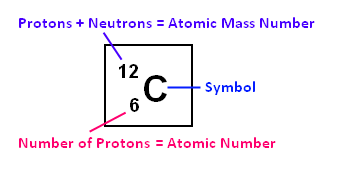Don’t let the numbers confuse you, they are just telling you how many electrons, protons and neutrons there are in an atom of Carbon.

Atomic Mass Number

This number tells you how many protons + neutrons there in an atom. This is always the biggest of the two numbers (unless they are the same). This can be remembered by ‘The MASS number is MASSive.’

Atomic Number

This tells you how many protons there are in an atom. (Remember, for just a normal atom, there are the same amount of protons as there are electrons).

Example Question:

How many protons, neutrons and electrons are there in an atom of Carbon?

6 protons (atomic number = 6)

6 electrons (atoms have the same amount of electrons and protons)

6 neutrons (mass number is 12 which is the neutrons + protons. There are 6 protons so there must be 6 neutrons left! 12 - 6 = 6.

## Isotopes

You will need to know:

What an isotope is.

How to find the ‘relative atomic mass’ of an element.

Isotopes are different types of the same element, which have the same amount of protons but a different amount of neutrons. Isotopes of the same element have the same atomic number but a__ different mass number.__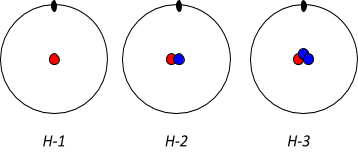Here are 3 different isotopes of Hydrogen, they can be known as H-1, H-2 and H-3.

Notice the number of protons and electrons are the same but the number of neutrons changes!

Many elements have more than one stable isotope (meaning they don’t fall apart), for example, Boron can exist as B-11 or B-10 - this means that Boron can have have a relative mass of 10 or a mass of 11.

You will be asked to find the relative atomic mass of isotopes. The equation below can be used (this is just a fancy-pants average):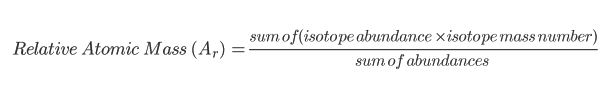Note: ‘abundance’ is a scientific way of saying ‘amount’.

You will come across a certain kind of question, which may seem difficult at first, but stick with it, once you know how to do one, you know how to do them all!

Let’s say we had collected all the Boron in the UNIVERSE, and 20% of it is B-10 and 80% of it is B-11. We can’t put two numbers for mass on the periodic table, sow we need to find an average mass. Since it is not split 50% 50%, we need to put it into a more complicated equation. Here is how to find the relative atomic mass of the Boron: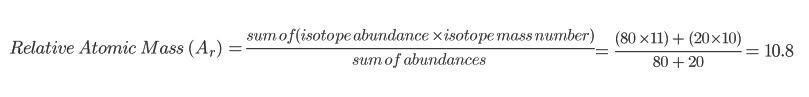Note: The answer is ALWAYS somewhere between the mass numbers of the isotopes.

How many protons, neutrons and electrons are in an atom of Sodium?